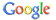web uni-protokolle.de powered by# Allgemein

Bücher > Mathematik > Stochastik > Statistik > AllgemeinNumerical Recipes in C++von William H. PressTheory of Stochastic Canonical Equations - Volumes I and II (MATHEMATICS AND ITS APPLICATIONS Volume 535)von Vyacheslav L. GirkoSymbolic Computation, Number Theory, Special Functions, Physics, and Combinatorics (Developments in Mathematics, 4)von Frank GarvanStatistics for Environmental Engineers, Second Editionvon Paul Mac BerthouexUnderstanding Design of Experiments : A Primer for Technologists (Hanser Understanding Books)von R. J. Del VecchioPrinciples and Practice of Structural Equation Modelingvon Rex B. KlinePrinciples and Practice of Structural Equation Modelingvon Rex B. KlineObject Orientated Implementation of Numerical Methodsvon Didier BessetA Primer on Regression Artifactsvon Donald Thomas CampbellSix Sigma and Beyond: Foundations of Excellent Performance, Volume 1von Dean H. StamatisA Primer of Probability Logic (Csli Lecture Notes, Number 68)von Ernest W. AdamsA Primer of Probability Logic (Csli Lecture Notes, No 68)von Ernest W. AdamsStochastic and Global Optimization (Nonconvex Optimization and Its Applications, 59)von Gintautas DzemydaApplied Nonparametric Statistical Methods, Third Editionvon Peter SprentClassification (Monographs on Statistics and Applied Probability, 82)von A. D. GordonAnalysis of Financial Time Seriesvon Ruey S. TsayTime Series Analysisvon James D. HamiltonEssentials of Statistics for Business and Economics with Data Files CD-ROMvon David Ray AndersonThe Elements of Statistical Learningvon Trevor HastieStatistikvon Joachim Hartung
Zum Thema:

HTML-Code zum Verweis auf diese Seite:
<a href="http://www.uni-protokolle.de/buecher/kat/520000/"> Allgemein </a>